# celestial coordinates

## celestial coordinates

[sə′les·chəl kō′ȯrd·nəts]
(astronomy)
Any set of coordinates, such as zenithal distance, altitude, celestial latitude, celestial longitude, local hour angle, azimuth and declination, used to define a point on the celestial sphere.

## Celestial Coordinates

(religion, spiritualism, and occult)

Celestial (sky) coordinates are most easily understood by comparing them to terrestrial (earthly) coordinates. Terrestrial coordinates allow a location to be specified in terms of degrees of longitude and latitude. Celestial coordinates similarly specify location in terms of two measurements of distance, expressed in terms of degrees of a circle. Rather than measuring degrees along the surface of planet Earth, however, celestial coordinates are measured against the celestial sphere, which is the sphere created by imagining that all of the objects in the sky are stuck against the inside of a gigantic, hollow sphere, with Earth located at the exact center.

There are several systems of celestial coordinates. The altitude-azimuth system begins from any given location on the Earth’s surface, and uses the horizon, the zenith, and the north-south axis as points of reference. The equatorial system uses the celestial equator and the vernal point as its primary reference points. Astronomers most often use this system. The ecliptic system uses the ecliptic and the vernal point. Astrologers most often use the ecliptic system.

## Celestial Coordinates

numbers used to specify the position of celestial objects and auxiliary points on the celestial sphere. Various systems of celestial coordinates are used in astronomy. Each is essentially a system of polar coordinates on a sphere with an appropriately chosen pole. A celestial coordinate system is given by a great circle on the celestial sphere or by its pole, which is situated at 90° from every point of this circle, together with the designation on the circle of the origin for measuring one of the coordinates. Celestial coordinate systems are called horizon, equatorial, ecliptic, or galactic systems, depending on the choice of this great circle. Celestial coordinates were already in use in remote antiquity. Several systems were described by the ancient Greek geometer Euclid (c. 300 B.C.). Hipparchus’ star catalog, which was published in Ptolemy’s Almagest, contained the positions of 1, 022 stars in the ecliptic coordinate system.

In the horizon coordinate system, the primary great circle is the mathematical, or true, horizon NESW (Figure 1), and the pole is the zenith Z of the point of observation. In order to specify the position of á celestial object σ, we draw a great circle through σ and Z, which is called the vertical circle of the object. The arc Zo- along the vertical circle from the zenith to the object is called its zenith distance Z and is the first coordinate; Z may have any value from 0° for the zenith Z to 180° for the nadir Z’. Instead of z, one can use the altitude h of the object, which is equal to the arc of the vertical circle from the horizon to the object. The altitude is measured in both directions from the horizon from 0° to 90° and is positive if the object is above the horizon and negative if the object is below the horizon. With this convention, the relation Z + h = 90° always holds.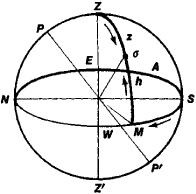Figure 1. Horizon celestial coordinate system

The second coordinate, the azimuth A, is the arc measured eastward from the north point along the horizon to the object’s vertical circle. (In astrometry, the azimuth is often measured westward from the south point S.) This arc, NESM, measures the spherical angle at Z between the celestial meridian and the object’s vertical circle; this spherical angle equals the dihedral angle between the two planes. The azimuth can have any value from 0° to 360°. An important characteristic of the horizon coordinate system is its dependence on the point of observation, since the zenith and the horizon are determined by the direction of the plumb line (vertical), which is different at different points on the earth’s surface. Because of this, the coordinates of even a very distant object are different when observed simultaneously from various points. In the process of moving along a diurnal circle, each object intersects the meridian twice; each passage of the object through the meridian is called a culmination. At the upper culmination, Z is smallest, and at the lower culmination, Z is largest. In the course of a day, Z varies between these limits. For objects whose upper culmination is south of Z, the azimuth A varies from 0° to 360° in the course of a day. But for objects that culminate between the celestial pole P and Z, the azimuth varies within certain limits that are determined by the latitude of the point of observation and the angular distance between the object and the celestial pole.

In the first equatorial coordinate system, the primary circle is the celestial equator QγQ1 (Figure 2), and the pole is the celestial pole P that is visible from a given point. In order to specify the position of an object σ, we draw a great circle, called the hour circle, through the object and through P. The arc of this circle from the equator to the object is the first coordinate—the object’s declination δ. The declination δ is measured from the equator in both directions from 0° to 90° and is taken to be negative for objects in the southern hemisphere. Sometimes, instead of the declination, we use the polar distance p, which is equal to the arc Per of the hour circle from the north celestial pole to the object; the quantity ρ can assume any value from 0° to 180°, so that the relation ρ + δ = 90° always holds.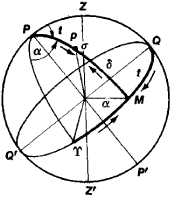Figure 2. First and second equatorial celestial coordinate systems

The second coordinate, the hour angle t, is the arc QM on the equator, measured from the intersection of the equator with the celestial meridian at the point Q located above the horizon in the direction of the rotation of the celestial sphere to the object’s hour circle. This arc corresponds to the spherical angle at P between the object’s hour circle and the arc of the meridian that is directed toward the south point. The hour angle of a fixed object changes from 0° to 360° in the course of a day, while the declination of the object remains constant. Since the hour angle changes in direct proportion to the time, it serves as a measure of time. The hour angle is almost always expressed in hours, minutes, and seconds of time; thus, 24 hr corresponds to 360°, 1 hr corresponds to 15°, and so forth. Both of the systems described above—the horizon and first equatorial systems—are called local systems, since coordinates in these systems depend on the point of observation.

The second equatorial coordinate system differs from the first only in the second coordinate. Instead of the hour angle, the second system uses the object’s right ascension α—the arc γ M on the celestial equator measured from the vernal equinox γ to the object’s hour circle in the direction opposite to the rotation of the celestial sphere (Figure 2). This arc measures the spherical angle at P between the hour circle passing through the point T and the hour circle through the given object. Usually α is expressed in hours, minutes, and seconds of time, and it may assume any value from 0 hr to 24 hr. Since the point γ rotates along with the celestial sphere, both coordinates of a sufficiently distant, fixed object in this system are independent of the point of observation.

In the ecliptic coordinate system, the primary circle is the ecliptic EγE’ (Figure 3), and the pole is the ecliptic pole Π. To specify the position of an object σ, we draw a great circle through the object and the point Π. This great circle is called the latitude circle of the given object, and the arc along it from the ecliptic to the object is called the ecliptic, celestial, or astronomical latitude β and is the first coordinate. The latitude β is measured from the ecliptic toward the north or south ecliptic poles; in the latter case, the value is taken to be negative. The second coordinate is the ecliptic, celestial, or astronomical longitude λ —the arc γM along the ecliptic from the point T to the object’s latitude circle, measured in the direction of the sun’s annual motion. It can have any value from 0° to 360°. The coordinates β and λ of points on the celestial sphere do not change in the course of a day and do not depend on the point of observation.

In the galactic coordinate system, the primary circle is the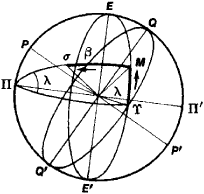Figure 3. Ecliptic celestial coordinate system

galactic equator BDB’ (Figure 4), which is the great circle on the celestial sphere that is parallel to the plane of symmetry of the Milky Way as seen from the earth; the pole is the pole Γ of this great circle. The position of the galactic equator on the celestial sphere can be determined only approximately. It is usually specified by the equatorial coordinates of its north pole, which are taken as α = 12 hr 49 min and δ = + 27.4° (epoch 1950.0). To specify the position of an object σ, we draw a great circle, called a galactic latitude circle, through the object and through the point Γ. The arc of this circle from the galactic equator to the object, called the galactic latitude b, is the first coordinate. The galactic latitude may have any value from +90° to —90°; the minus sign corresponds to galactic latitudes of objects in the hemisphere in which the south celestial pole is located. The second coordinate, the galactic longitude l, is the arc DM measured along the galactic equator from the point D at which the galactic equator intersects the celestial equator to the object’s galactic latitude circle; the galactic longitude / is measured in the direction of increasing right ascension and may assume any value from 0° to 360°. The right ascension of the point D is equal to 18 hr 49 min. Coordinates in the first three systems are determined from observations that are made by using appropriate instruments. Ecliptic and galactic coordinates are obtained from the equatorial coordinates by means of calculations.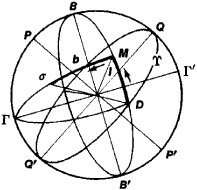Figure 4. Galactic celestial coordinate system

In order to compare the celestial coordinates of objects observed from different points on the earth or at different times of the year (at different points on the earth’s orbit), these coordinates are referred either to the center of the earth or to the center of the sun, with parallax being taken into account. Because of precession and nutation, there is a slow change in the orientation in space of the plane of the celestial equator and the plane of the ecliptic, which define the primary circles in a number of celestial coordinate systems; the origins from which the coordinates are measured also change. As a result of this, the values of the celestial coordinates also change slowly. Therefore, in order to specify the precise location of objects on the celestial sphere, we must indicate the moment of time (the “epoch”), which determines the position of the celestial sphere and the ecliptic. The position of objects in a given celestial coordinate system proves to be affected by the aberration of light, which is a consequence of the earth’s orbital motion (annual aberration) and the observer’s motion owing to the earth’s rotation (diurnal aberration); the position is also affected by the refraction of light in the atmosphere. The celestial coordinates of an object also change as a result of the proper motion of the object.

Observations of variations in celestial coordinates have led to very significant discoveries in astronomy, which are of great importance for the understanding of the universe. These discoveries include the phenomena of precession, nutation, aberration, parallax, and of the proper motion of stars. Celestial coordinates make it possible to solve the problem of measuring time and the determination of geographic coordinates of different points on the earth’s surface. Celestial coordinates find wide application in the compilation of various star catalogs, in the study of the true motions of celestial bodies (both natural and artificial) in celestial mechanics and in astrodynamics, and in the study of the spatial distribution of stars in stellar astronomy.

### REFERENCES

Blazhko, S. N.Kurs sfericheskoi astronomii. Moscow-Leningrad, 1948.
Kazakov, S. A.Kurs sfericheskoi astronomii, 2nd ed. Moscow-Leningrad , 1940.
V. P. SHCHEGLOV

## celestial coordinates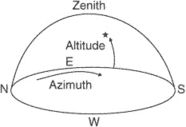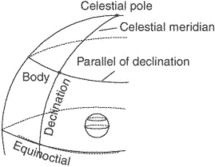Equinoctial system of celestial coordinates.
Two coordinates on the surface of the celestial sphere used to determine the position of a celestial body. Two sets of celestial coordinates are in common use: the equinoctial system, in which the coordinates are a declination and an hour circle, and the horizon system, in which the coordinates are a vertical circle passing through the given celestial body and an altitude circle.
References in periodicals archive ?
The name of the newly discovered pulsar-J1838-0537 -- comes from its celestial coordinates.
Like a 21st-century greatest hits from Burnham's Celestial Handbook, Stars & Planets profiles each of the constellations and highlights its major stars and deep-sky objects, noting their celestial coordinates and a picture or two of the most photogenic.
1090 and has several interchangeable plates, each engraved with the celestial coordinates for a different latitude.
Such was a practice of mapping since Ptolemy, because both depended on the same mathematical skills and the same celestial coordinates.
modify and clean up data that has instrument noise, such as stray photons or cosmic ray glitches -- look at celestial objects in 3D -- plot surfaces -- measure alignment between images, to compare the movement of images in the same part of the sky on multiple days -- measure brightness -- apply filtering to highlight specific data -- turn pixel coordinates into real celestial coordinates, so students can learn standard measurement terminology and communicate with professional astronomers -- annotate with arrows, circles, text -- save and print -- output as a jpeg, png, and FITS (Flexible Image Transport System), the standard for transmitting astronomical data
Voyager 4's Coordinate panel provides the cursor's celestial coordinates as it moves around the display.
Celestial coordinates are given by the ticks along the sides.
There's a long list of popular targets that will be visible at the selected time, but you can also choose a faint fuzzy from among a dozen major catalogs or enter your own celestial coordinates.
4 for its celestial coordinates in Leo (or SDSS 1035 for short), turned out to be an eclipsing binary with an orbital period of 82 minutes.
I use Burnham's Celestial Handbook, which gives objects' celestial coordinates for equinox 1950.
Knowing the approximate celestial coordinates of the Moon and the local sidereal time, I could calculate that the Moon was roughly overhead at latitude 16[degrees] N, longitude 24[degrees] W.

Site: Follow: Share:
Open / Close